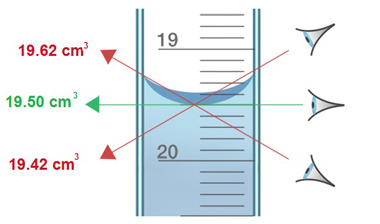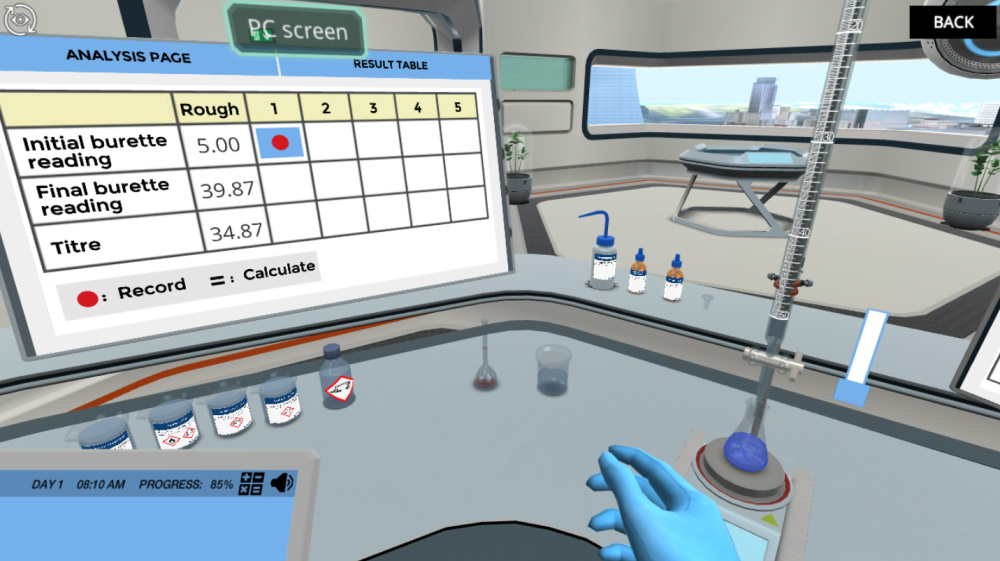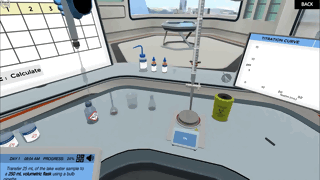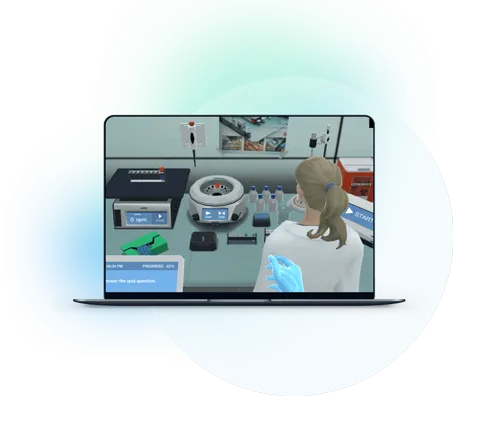Blog

# 5 Interesting Ways of Teaching Titration to Students

Teaching with Labster
October 27, 2022

Titration is a technique to measure the concentration of an acid or alkali in a sample by comparing it to the concentration of an alkali or acid in a standard solution. There are two main types of titration rough titration and accurate titration. Rough titration is the first titration, and it is used to get an approximation volume needed to reach the end-point. And the accurate titration is carried out after the rough titration is performed. Accurate titration is performed carefully until the concordant titer is achieved.

# Why do students struggle to understand titration?

### 1. Lack of basic knowledge

If the students lack basic knowledge about titration, they will face difficulties while experimenting. So first, find out the level of their basic information and clear their doubts. Explain to them the main purpose of titration, that we perform titration to determine the quantity of any compound by using a measured quantity of another compound. Explain the basics first and also describe how an indicator works.

### 2. The readings and calculations

In titration, the correct readings are necessary. Students face difficulties mostly in burette reading; show them how to read a burette correctly. In burette reading, the human eye's position is very important; make sure your eye is at the same level as the meniscus to avoid an error. The meniscus is concave or like a U shape for water and most liquids. Read the center of the meniscus or the bottom of the U-shape. The second thing in the unit conversions in the calculations, the students must know how to convert the units.

### 3. It’s conceptually challenging.

Theoretical imagination of the titration is complicated, as this process involves a color change process. This may confuse the students on how and why the color changes and the reagents' colors. So video graphics is a better option when you don't have the apparatus. You can use your laptop to show the images or draw the apparatus and the procedure, which is more time-consuming.

# 5 interesting ways of exploring titration

### 1. Review the basics of titration

• Rough titration: A rough titration is the first titration, and it is used to get an approximation volume that is needed to reach the end-point.

• Accurate titration: Accurate titration is carried out after the rough titration. Accurate titration is performed carefully until the concordant titer is achieved.

• Concordant titres: "concordant titres" refers to the volume of two or more titres that are similar in quantity.

• Equivalence point: An equivalence point is the theoretical completion of the reaction. It means the number of moles of the base is equal to the number of moles of acid.

• Indicator: a dye or mixture of dye that marks the end-point of the titration.

• Titre: the total volume of solution added to reach the end-point of the titration.

• Standard solution: a solution in which the concentration of solute in mol dm-3 is known accurately.

• Acid-base indicator: An acid-base indicator is a dye or mixture of dye that marks the end-point of the titration. The indicator has a sudden and obvious color change at the pH corresponding to the end-point of the titration.

• Balanced chemical equation: In a balanced equation, the total number of atoms of each kind (e.g., A) in the reactants and products is the same.

• End-point: The end-point is a physical change in the solution, as determined by the indicator. The endpoint is not necessarily the same as the equivalence point, even though both represent the same idea (the completion of titration).

## 2. Briefly explain the titration apparatus

The major apparatus used in titration are:

• Burette: measures the volume of an added solution accurately.

• Bulb pipette: delivers an accurate volume of a solution.

• Volumetric flask: makes up an accurate volume of a solution.

Before their use, the burette and pipette are cleaned and rinsed with the solution they will eventually contain. Rinsing with water or other solution may change the concentration of the solution that the burette and pipette will eventually contain.

### How to read the burette

• Make sure your eye is at the same level as the meniscus to avoid an error. The meniscus is concave or like a U shape for water and most liquids. Read the center of the meniscus or the bottom of the U-shape.Figure 1: How to read the meniscus. The correct reading is 19.50 mL. Due to parallax error, the reading can be 19.62 mL or 19.42 mL (Image source Labster theory).

• The first and final reading of the burette should be recorded. The difference between the first and final reading is called the titer.

• Readings should be recorded to the nearest 0.05 mL. Examples of readings: 22.05, 23.00, and 23.05 mL.

A volumetric flask is used to prepare a chemical solution with an accurate and precise volume.

#### How to use a volumetric flask:

• Measure and add the solute to the solution.

• Add enough solvent to dissolve the solute.

• Continue to add solvent until you reach the line marked on the volumetric flask.

• Seal the volumetric flask and invert it to thoroughly mix the solution.

## 3. Explain the procedure for titration (Neutralize an acid lake contamination)

The procedure of titration involves three main steps; preparation of solutions, performing titration, and the result analyses.

### Prepare the solutions

• Place the unknown concentration solution in a conical flask using a calibrated pipette.

• Add a few drops of indicator into the unknown concentration solution.

• Fill a clean burette with the standard solution.

### Perform titration

• Record the volume of the solution in the burette (First reading).

• The solution should be added dropwise from the burette while continually swirling the flask when the end-point is close.

• Record the volume of the solution in the burette (Final reading).

### Prepare for result analysis

• Repeat titration until concordant titres are achieved.

• Calculate the average of the concordant titres.## 4. Explain the result analysis

### Finding the concentration of a solution

We require the following information to determine the concentration of a solution:

• The balanced equation for the reaction

• The volume of the solution with an unknown concentration in the conical flask

• The volume of standard solution in the burette

• The concentration of the standard solution in the conical flask

#### Work example

An acid-base titration is performed to find the concentration of sodium hydroxide. 50.0 mL of a solution of sodium hydroxide is neutralized by exactly 24.50 mL of hydrochloric acid of concentration 0.250 mol/L.

NaOH + HCl → NaCl + H₂O

Calculate the concentration, in mol/L, of the sodium hydroxide solution.

What we know:

Reaction: NaOH + HCl → NaCl + H₂O

Step 1. Calculating the moles of acid

Moles = concentration (mol/L) x volume of solution (mL) = 0.25 x 24.5/1000 = 0.006125 mol

Step 2. Calculating the moles of base

Use the stoichiometry of the balanced equation to calculate the moles of NaOH. The balanced equation shows that 1 mol of NaOH reacts with every 1 mol of HCl.

Moles of NaOH = moles of HCL = 0.006125 mol

Step 3. Calculating the concentration of base after dilution (10x)

Concentration (mol/L) = number of moles of solute (mol)/ volume of solution = 0.006125 /(50/1000) = 0.123 mol/L

Additional step. Calculating the concentration of base before dilution (if the sample was diluted)

Final concentration (mol/L) = concentration (mol/L) x dilution factor = 0.123 x 10 = 1.23 mol/L

## 5. Use virtual lab simulations

With technological advances, it is much easier to explain complex and challenging processes with the help of simulations. Now, you can simulate experiments without the need for any valuable equipment. In this regard, you can take help from Labster’s virtual lab simulations. These simulations engage students through interactive learning scenarios. Students dive into a 3D world, where they visually learn and apply their concepts to solving real-life problems.In Labster’s interactive Titration: Neutralize an acid lake contamination Virtual Lab, you will embrace the power of the titration technique. With the appropriate lab apparatus, a chemical indicator, and a base solution, you can determine the concentration of any acid. We will help you ace the assembly process so that once you go to the real-life lab, you will be able to Recognize and explain the function of each part of the apparatus used in the titration technique.What exactly are virtual labs?